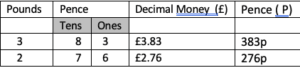Handling money and learning the maths behind calculating with money is a useful area that parents can cover at home. The tool required is real money   even better than  the ‘pretend’ money used in primary schools.

Pre–skills

To enable a full appreciation of money, the learner needs to be able to count in ones, twos, fives, and tens as these are required for handling our coins and notes. We have 1p, 2p, 5p,  10p, then we have to calculate in tens, 20p, 50p, then pounds £1,  and £2 coins,  then in notes £5, £10, £20, and £50.

Steo 1

Identify the different coin values. Initially 1p, 2p, 5p, 10, 20, and 50p. Take particular care as the newest coins no longer have numerals on them, only the number word, ie the word twenty rather than the number 20.

Sort a pile of coins into the different values.

Step 2

Match these piles to cards with 1p 2p 5p etc written on them. They will begin to understand the symbols we use to represent the coins.

Step 3

Find equivalent values in single pence for 2p, 5p, 10p, 20p,

eg 2p = 1p +1p   5p = 1p+1p+1p+1p+1p

Step  4

Use 2p coins to find equivalent values for 5p (2p+2p+1p), 10p and 20p

(Running out of the necessary coins might be a problem so another useful  activity relaring to money could be to make some paper coins from the real money – use a ‘brass rubbing’ approach with a pencil over real coins and then cut them out. The coins can be rubbed on both sides and stuck together. This sort of very practical activity engages the child, is fun and they remember it!).

Step 5

Use 5p coins to find equal values for 10p, 20p 50p

Step 6

Use 10p coins to find equal values for 20p 50p and introduce the £1

Step 6

Use 20p and 50p to find equal values for £1

Step 7

Pound coins and notes can be added one at a time and a similar approach to equivalent pound coins to note values explored. Paper notes can be artistically recreated or redesigned using the child’s own heroes –  eg Spiderman or Elsa on the £5 note.

Step 8

Put all coins  in a bag,  the child puts their hand in and draws out a handful. Count up the total. Make it a game. The winner is the one with the highest total.  A good lesson is learnt when it is realised that more coins is not necessarily a bigger total and the value of each coin is of greater importance.

The following grid is a useful way of recording that total.Explain the decimal point in writing money, it  may be their first experience of the point separating the whole (pounds) from the parts of a pound (pence).

Step 8

Choose coins in response to a request for a certain amount.

(This is where a ‘pretend’ shop set up at home is great fun. It could be a toy or food shop with the relevant price tags.)

Step 9

Give change. If I have spent 56p then I need 44p change from £1. Counting on from the 56p to the pound can be easier  (2 x 2p to 60p then 20p to 80p and another 20p to £1.

Step 10

None of these activities has required any workbooks or worksheets but further activities involving problem solving using money may need a paper and pencil.

These problems are best devised using familiar items and initially with the support of the physical money created  – eg. How much would 5 apples cost if one apple costs  30p. What coins would I need?